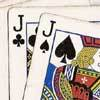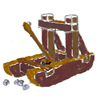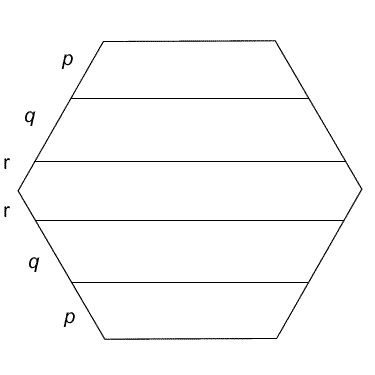#### You may also like### How Big?

If the sides of the triangle in the diagram are 3, 4 and 5, what is the area of the shaded square?### Do Unto Caesar

At the beginning of the night three poker players; Alan, Bernie and Craig had money in the ratios 7 : 6 : 5. At the end of the night the ratio was 6 : 5 : 4. One of them won $1 200. What were the assets of the players at the beginning of the evening?### Oh for the Mathematics of Yesteryear A garrison of 600 men has just enough bread ... but, with the news that the enemy was planning an attack... How many ounces of bread a day must each man in the garrison be allowed, to hold out 45 days against the siege of the enemy? # Hexagon Slices ##### Age 11 to 14 Short Challenge Level: This regular hexagon has been divided into four trapezia and one hexagon. If each of the five sections has the same perimeter, what is the ratio of the lengths$p$,$q$and$r\$?If you liked this problem, here is an NRICH task which challenges you to use similar mathematical ideas.

This problem is taken from the UKMT Mathematical Challenges.
You can find more short problems, arranged by curriculum topic, in our short problems collection.